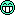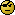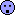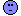BE-proff
★★

Russia,
2019-02-04 12:12

Posting: # 19855
Views: 826

## Formulas to calculate sample size [Power / Sample Size]

Hi All,

Recently I have started learning Python and I'd like to write script calculating sample size (the same as sampleN.TOST function in R).Can anybody share formula of formulas set for relevant calculations?ElMaestro
★★★

Denmark,
2019-02-04 13:27

@ BE-proff
Posting: # 19857
Views: 684

## Formulas to calculate sample size

Hi BE-proff,

» Can anybody share formula of formulas set for relevant calculations?This is a great challenge you awarded yourself.Sample size calculation is not a set of deterministic equations (yet?!?), but usually involve integrals.

For a start try and look at Potvin's paper.
Here you'll find a very decent approximation which is based on the central t test. This provides the "exact" solution when the expected match is 100% and it is a very decent* approximation in most other relevant case whenever desired power is above the magical 80%. Perhaps you can start there and then make it more complicated and exact once you get into it?

Here's how I would approach it (and I am assuming you are not relying on external libraries**):
1. Make a function for the density of central t at any level of df.
2. Make a function that can integrate it, from minus infinity to some arbitrary value x.
3. Make a function that can find the critical value of the central t for an arbitrary p-level between 0 and 1. This one may be a little tricky if you write it from scratch but you can find inspiration by googling Russel Lenth's ASA243 Al Gore Rhythm. I imagine you can also find some py code on the www but I am not certain about it.

With those three at hand you can reproduce power.TOST sand sampleN.TOST values using the central t approximation by insertion into the equation from Potvin. You may be able to find a way to import and use gsl or similar libraries in python, then it is simply a matter of calling some functions. Can't help with the implementation of it as I don't do python.

Next, if you are hardcore you can look into noncentral t and Owen's Q. If you can write the framework above then you have all the skill at hand to also do this last step.
This will give you the opportunity to get anything power.TOST does.

Happy coding. Interested to hear of your further achievements, so please keep me posted.

* I am sure the BE police will spank me for saying so but I am willing to defend my words.** I am also willing to at least read posts asking why someone would want to reinvent the wheel.``` if (3) 4 x=c("Foo", "Bar") b=data.frame(x) typeof(b[,1]) ##aha, integer? b[,1]+1 ##then let me add 1```

Best regards,
ElMaestro

“(...) targeted cancer therapies will benefit fewer than 2 percent of the cancer patients they’re aimed at. That reality is often lost on consumers, who are being fed a steady diet of winning anecdotes about miracle cures.” New York Times (ed.), June 9, 2018.
BE-proff
★★

Russia,
2019-02-04 13:43

@ ElMaestro
Posting: # 19858
Views: 656

## Formulas to calculate sample size

Hi ElMaestro,

Thank you for clarification.
It looks really very challenging...So I can't promise you quick response - wait for some time.ElMaestro
★★★

Denmark,
2019-02-04 17:17

@ BE-proff
Posting: # 19864
Views: 638

## Hey, python is not so difficult :p

Hi BE-proff,

I just downloaded python and played around with it.
Syntatically it is not so hard, I think.

Below are some functions that will get you started. They execute just fine as a script on my computer (Win10). The functions use Simpson integrals and various constants that that you can play around with to achieve the combination of accuracy and speed that suits you. Note that I only made step 1-3, so I will leave it to you to put these into Potvin's equation.
They are in no way optimized so there's plenty of work to do still``` import math def GammaB(z):  x=0.0  dx=0.04  integral=0.0  di=10  it=0  while ((di>0.001) or  (x<8*z)):   y =pow(x, z-1)*math.exp(-x)   y1=pow(x+dx, z-1)*math.exp(-(x+dx))   y2=pow(x+dx+dx, z-1)*math.exp(-(x+dx+dx))   di= (dx/3)*(y+4*y1+y2)   integral=integral +di   x=x+dx+dx   it+=1  return(integral) def DensityB(x, df):  a=GammaB((df+1)/2.0 )  b=math.sqrt(df*math.pi) * GammaB(df/2.0 )  c=math.pow(1.0+x*x/df, -( (df+1) / 2.0))  return(a*c/b) def probtcum(df, t): ## note: for t>0 only, you can easily fix it for negative t  x=0.0  integral=0.5  dx=0.5*t/100  i=0  while (i<100):   y=DensityB(x, df)   y1=DensityB(x+dx, df)   y2=DensityB(x+dx+dx, df)    di = (dx/3)* (y+4*y1+y2)   integral=integral+di   x=x+dx+dx   i+=1        return(integral) def critvalt(df, p): ##fix it yourself for p lowe than 0.5x=0.0  integral=0.5  dx=0.004  while (integral<p):   y=DensityB(x, df)   y1=DensityB(x+dx, df)   y2=DensityB(x+dx+dx, df)    di = (dx/3)* (y+4*y1+y2)   integral=integral+di   x=x+dx+dx  ##aha!! now the solution is between x and x-2dx  ##so we can just interpolate linearly  a=di / (dx+dx)  b=integral-a*x  soln=(p-b) /a  return(soln) ## in R, pt(df=5, 0.4) is 0.6471634 p=probtcum(5, 0.4) print("probt cumul at df=5 for x=0.4", p, "should be", 0.6471634) ## in R, qt(df=11, 0.95) is 1.795885 q=critvalt(11, 0.95) print("critt at df=11 and p=0.95=", q, "should be", 1.795885) ```

On my machine I get:

``` >>> RESTART: [blahblah] probt cumul at df=5 for x=0.4 0.6471629404086429 should be 0.6471634 critt at df=11 and p=0.95= 1.7958969293454883 should be 1.795885 >>> `````` if (3) 4 x=c("Foo", "Bar") b=data.frame(x) typeof(b[,1]) ##aha, integer? b[,1]+1 ##then let me add 1```

Best regards,
ElMaestro

“(...) targeted cancer therapies will benefit fewer than 2 percent of the cancer patients they’re aimed at. That reality is often lost on consumers, who are being fed a steady diet of winning anecdotes about miracle cures.” New York Times (ed.), June 9, 2018.
ElMaestro
★★★

Denmark,
2019-02-04 22:31

@ BE-proff
Posting: # 19865
Views: 550

## experiments with a function optimization

Hey,

learning python is great funThe code below is executing quite instantaneously on my machine.
It involves a simple re-write of the density function using the very handy shortcut noted on wikipedia.

``` import math def DensityC(x, df):     if ((df % 2) ==0):        q=df-1        Den=2*math.sqrt(df)        Num=1        while (q>=3):            Num = Num*q            Den = Den*(q-1)            q=q-2        x=(Num/Den)*math.pow(1.0+x*x/df, -( (df+1) / 2.0))     if ((df % 2) ==1):        q=df-1        Den=math.pi*math.sqrt(df)        Num=1        while (q>=1):            Num = Num*q            Den = Den*(q-1)            q=q-2        x=(Num/Den)*math.pow(1.0+x*x/df, -( (df+1) / 2.0))     return(x) def probtcum(df, t): ## note: for t>0 only, you can easily fix it for negative t  x=0.0  integral=0.5  dx=0.5*t/100  i=0  while (i<100):   y=DensityC(x, df)   y1=DensityC(x+dx, df)   y2=DensityC(x+dx+dx, df)    di = (dx/3)* (y+4*y1+y2)   integral=integral+di   x=x+dx+dx   i+=1        return(integral) def critvalt(df, p): ##fix it yourself for p lowe than 0.5x=0.0  integral=0.5  dx=0.0004  while (integral<p):   y=DensityC(x, df)   y1=DensityC(x+dx, df)   y2=DensityC(x+dx+dx, df)    di = (dx/3)* (y+4*y1+y2)   integral=integral+di   x=x+dx+dx  ##aha!! now the solution is between x and x-2dx  ##so we can just interpolate linearly  a=di / (dx+dx)  b=integral-a*x  soln=(p-b) /a  return(soln) ## in R, pt(df=5, 0.4) is 0.6471634 p=probtcum(5, 0.4) print("probt cumul at df=5 for x=0.4: ", p, "should be", 0.6471634) ## in R, qt(df=11, 0.95) is 1.795885 q=critvalt(11, 0.95) print("critt at df=11 and p=0.95:     ", q, "should be", 1.795885) ```

Now don't feed it non-integer df's without extending the density function appropriately.

``` if (3) 4 x=c("Foo", "Bar") b=data.frame(x) typeof(b[,1]) ##aha, integer? b[,1]+1 ##then let me add 1```

Best regards,
ElMaestro

“(...) targeted cancer therapies will benefit fewer than 2 percent of the cancer patients they’re aimed at. That reality is often lost on consumers, who are being fed a steady diet of winning anecdotes about miracle cures.” New York Times (ed.), June 9, 2018.
BE-proff
★★

Russia,
2019-02-05 07:19

@ ElMaestro
Posting: # 19866
Views: 507

## experiments with a function optimization

Hi ElMaestro,

Star is shockedSince my math skills are not very strong I will hardly make out the ideaBut nevertheless I have managed to realize CVfromCI function in pytoBioequivalence and Bioavailability Forum |  Admin contact
19,478 posts in 4,133 threads, 1,333 registered users;
online 11 (0 registered, 11 guests [including 9 identified bots]).
Forum time (Europe/Vienna): 04:52 CEST

If you don’t know anything about computers,
just remember that they are machines that do exactly what you tell them
but often surprise you in the result.    Richard Dawkins

The BIOEQUIVALENCE / BIOAVAILABILITY FORUM is hosted byIng. Helmut Schütz